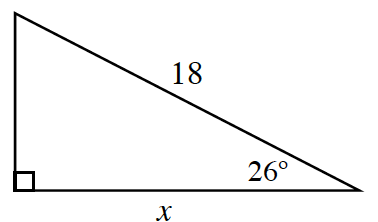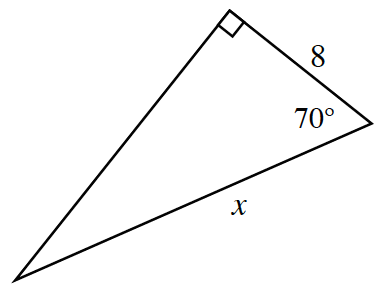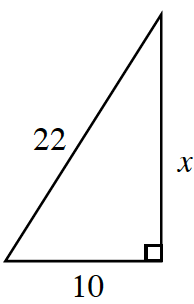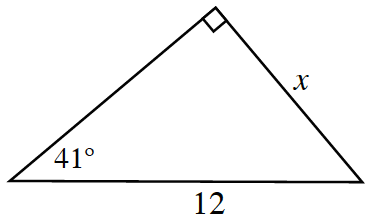### Home > PC3 > Chapter 1 > Lesson 1.1.4 > Problem1-39

1-39.

Solve for $x$ in each triangle below.

$\sin(\alpha) = \frac{\text{opposite}}{\text{hypotenuse}}$

$\cos(\alpha) = \frac{\text{adjacent}}{\text{hypotenuse}}$

$\tan(\alpha) = \frac{\text{opposite}}{\text{adjacent}}$

1.Label the marked sides as opposite, adjacent, or hypotenuse.

Write an equation involving the trigonometric ratio that corresponds to the given sides and angle.

$\operatorname { cos } ( 26 ^ { \circ } ) = \frac { x } { 18 }$

Solve for $x$. (Use your calculator.)

1.Follow the same steps in part (a).

1.$x\approx19.596$
1.Follow the same steps in part (a). Note that this time $x$ is the opposite side instead of the adjacent side.